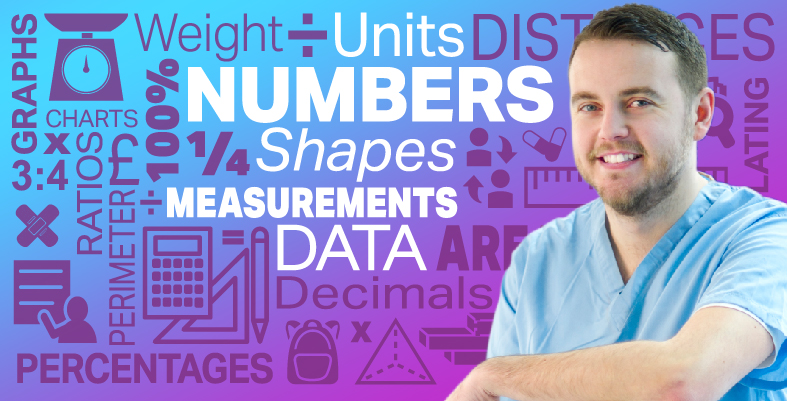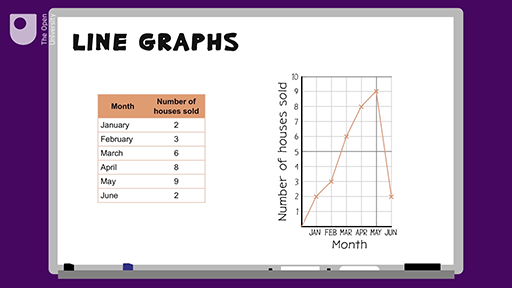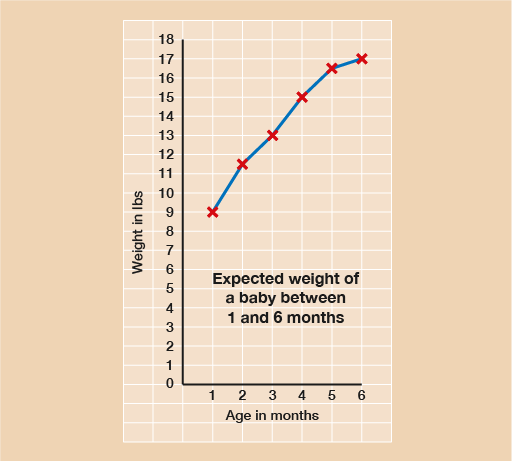Everyday maths for Health and Social Care and Education Support 1

Start this free course now. Just create an account and sign in. Enrol and complete the course for a free statement of participation or digital badge if available.

Free course

# 6 Line graphs

Now that you’ve had a look at pie charts and bar charts, let’s take a look at line graphs. These are drawn by marking (or plotting) points and then joining them with a straight line. You might have seen them used to show the expected growth patterns of a baby or young child.

Hint: It is best to use graph or squared paper when drawing line graphs because it makes it easier to plot the points.

## Example: The estate agent

How would you present information as a line graph? Watch the following video to find out.Skip transcript

#### Transcript

Here is a table showing the number of houses sold by an estate agent over a six-month period. How could you represent this information as a line graph?

To draw this in a graph, you need to first draw the axes. The months go on the bottom (horizontal) axis, and the house sales go up the left-hand side on the vertical axis.

Next, we need to decide on how we divide up these axes. There are six months, so there will be six marks on the horizontal axis. The highest number of house sales was 9, so the vertical axis will go from 0 to 10.

Now we can begin to mark our points. Starting with the first row of the table, you go up the line marked January, and then when you get to the line going across marked 2, you make a small cross. You then do this for the other points. Finally, you join the points using a ruler, and add your title.

Here’s a summary. To draw a line graph, you need to draw the horizontal and vertical axes and label them. Divide these axes into suitable scales to do this, you need to look at the data and find out what the smallest and largest numbers are. Plot the points from your data using a pencil to make small crosses. Join the points using a ruler, and give your graph a title.

End transcript

Interactive feature not available in single page view (see it in standard view).

Now try the following activity. Remember to check your answers once you have completed the questions.

## Activity 10: Creating a line graph

The following table shows the average weight of a baby from 1–6 months. Draw a line graph using the data from the table and then answer the questions below.

Age (months)Weight (lbs)
19
211.5
313
415
516.5
617
1. Between which two months is the greatest weight gain expected?
2. Between which two month is the least weight gain expected?

When drawing your line graph you should:

• draw the horizontal axis, labelling it ‘Age in months’, and the vertical axis, labelling it ‘Weight in lbs’
• divide these axes into suitable scales – your smallest and largest numbers are 9 and 17, so your scale could be one square for one pound (lb)
• plot the points from your data, using a pencil and make small crosses
• join the points using a ruler
• give your graph a title such as ‘Expected weight of a baby between 1 and 6 months’.

The finished line graph should look something like this:Figure 11 Weight of a baby line graph

1. The greatest expected weight gain is between 1 and 2 months when the baby’s weight is expected to increase by 2.5 lbs.
2. The least weight gain expected is between 5 and 6 months when the baby’s weight is only expected to increase by 0.5 lbs.

Self-check: always remember the following statements

Before moving on, you need to make sure you are able to collect, organise and show data in the forms of tables, diagrams, charts and graphs. Ask yourself the following questions:

• When I draw tables, diagrams, charts and graphs, is my data displayed clearly so that the information is easy to understand?
• Do I always include titles, scales, labels and keys when they are needed?

If you are not sure about these points, show your work to someone else and ask if they understand the data.

## Summary

In this section you have learned about how to present data in line graphs.

FSM_SSH_1